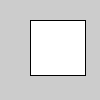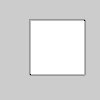# Py5Shape.is2d()#

Boolean value reflecting if the shape is or is not a 2D shape.

## Examples#def setup():
s = py5.create_shape()
s.begin_shape()
s.vertex(30, 20)
s.vertex(85, 20)
s.vertex(85, 75)
s.vertex(30, 75)
s.end_shape(py5.CLOSE)

py5.println(s.is2d(), s.is3d())
py5.shape(s)def setup():
py5.size(100, 100, py5.P3D)
s = py5.create_shape()
s.begin_shape()
s.vertex(30, 20)
s.vertex(85, 20)
s.vertex(85, 75)
s.vertex(30, 75)
s.end_shape(py5.CLOSE)

py5.println(s.is2d(), s.is3d())
py5.shape(s)


## Description#

Boolean value reflecting if the shape is or is not a 2D shape.

If the shape is created in a Sketch using the P3D renderer, this will be False, even if it only uses 2D coordinates.

Underlying Processing method: PShape.is2D

## Signatures#

is2d() -> bool


Updated on March 06, 2023 02:49:26am UTC